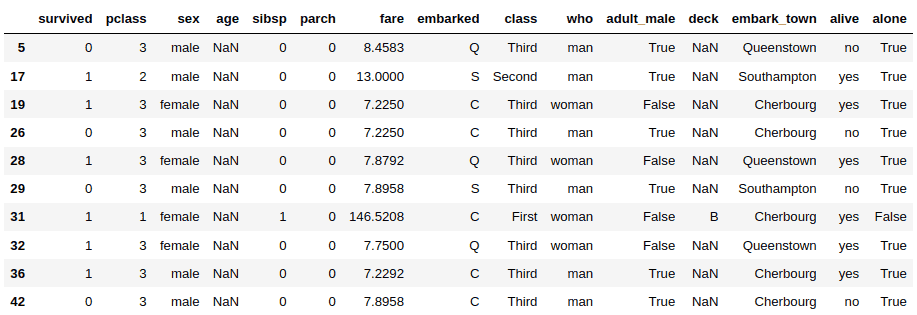Pandas DataFrames are data structures that store data in tabular form. Dataframes offer several functionalities for data manipulation, including sorting, grouping, merging and modifying.

This guide will show you everything you need to know about sorting Pandas DataFrames using column values, column names and index values. We’ll also show you what to do when you need to sort a Pandas DataFrame containing missing values.

## Sorting DataFrame by a Single Column values

To demonstrate dataframe sorting functionalities, you will be using the titanic dataset from the Seaborn library. The following script imports the titanic dataset:

``````import seaborn as sn

Output:You can use the `sort_values()` function to sort a Pandas dataframe by column values. All you need to do is pass the column name to the `sort_values()` function. For example, the following script sorts records in the titanic dataset by the “age” column.

``````temp_df = df.sort_values('age')

Output: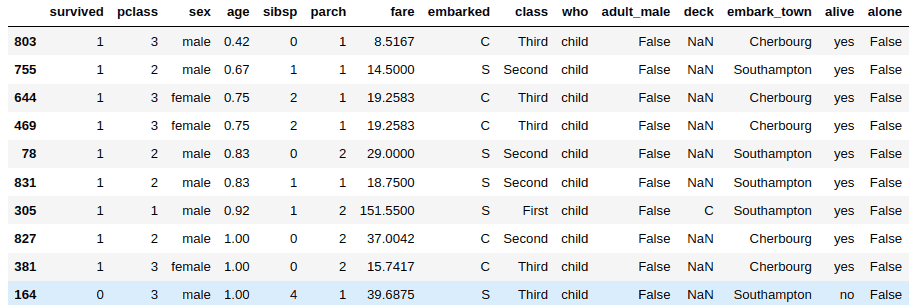By default, the records are sorted in ascending order. To sort a Pandas dataframe in descending order, you need to pass “False” as the value for the `ascending` parameter of the `sort_values()` function. Here’s an example.

``````temp_df = df.sort_values('age', ascending=False)

Output: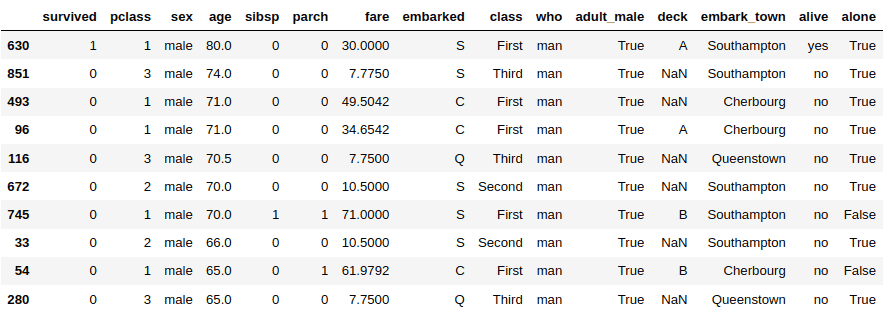To sort a Pandas DataFrame in place, you need to pass “True” as the value for the `inplace` parameter. This way you don’t have to store the result of your sorted dataframe in another dataframe.

``````df.sort_values('age',inplace = True)

Output:## Sorting DataFrame by Multiple Columns Values

You can sort a Pandas DataFrame by multiple column values. To do so, you need to pass a list of columns to the `sort_values()` function.

Let’s start clean and import the titanic dataset again.

``````import seaborn as sn

The following script sorts the pandas dataframe by the “age” and “fare” columns. The order of the column names in the list matters. The following script sorts the dataframe first by the “age” column and then by the “fare” column (in case the “age” column contains identical values), and it shrinks the DataFrame so it only contains those two columns. You can remove the trailing `[['age','fare']]` if you want to return the full DataFrame sorted by age and fare.

``````temp_df = df.sort_values(['age','fare'])[['age','fare']]

Output: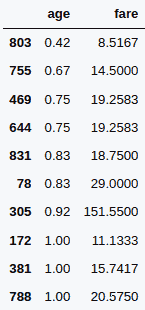Similarly, you can sort a dataframe by multiple columns in descending order. Like before, you need to pass “False” as the value for the `ascending` parameter, like we do here:

``````temp_df = df.sort_values(['age','fare'],
ascending=False)[['age','fare']]

Output: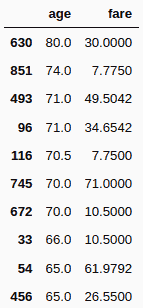I put together a Python Developer Kit with over 100 pre-built Python scripts covering data structures, Pandas, NumPy, Seaborn, machine learning, file processing, web scraping and a whole lot more - and I want you to have it for free. Enter your email address below and I'll send a copy your way.

Yes, I'll take a free Python Developer Kit

## Sorting Multiple Columns in a DataFrame with Different Sort Orders

You can specify different sorter orders for columns while sorting a dataframe by multiple columns, too, which is pretty powerful. You need to pass a list of boolean values corresponding to column names, to the `ascending` parameter. A value of “True” sorts a dataframe by ascending order, while the “False” value sorts a dataframe in descending order.

For example, the following script sorts the titanic dataset by the “age” column in the ascending order, and then by the “fare” column in descending order.

``````temp_df = df.sort_values(['age','fare'],
ascending=[True, False])[['age','fare']]

Output: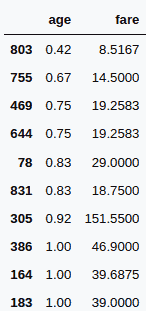## Sorting DataFrame by Index Values

You can sort a Pandas dataframe by its index values, too. A dataframe index is a list of (usually) numerical values that correspond to the locations of records in a dataframe.

When you sort a dataframe by a column value, the index values may become unsorted. For example the following script sorts the “titanic” dataset by the “age” column, just like we did earlier. In the output, you can see that the index values have become unsorted.

``````temp_df = df.sort_values('age')

Output:To sort a dataframe with unorganized index values, you can use the `sort_index()` function, as demonstrated in the following script:

``````temp_df = temp_df.sort_index()

Output: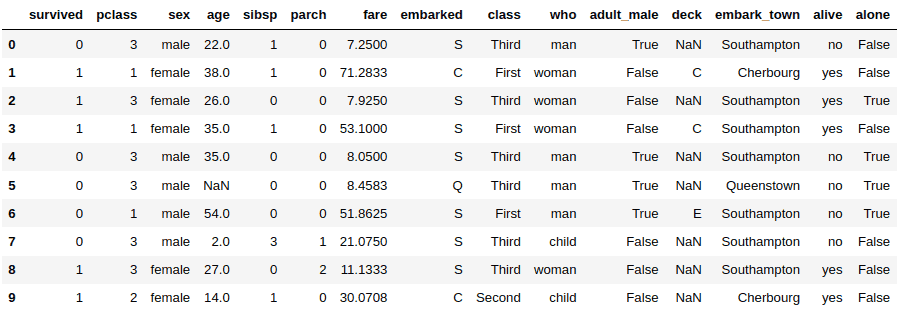You can sort a DataFrame in the descending order of the index values by passing a “False” value to the `ascending` parameter of the `sort_index()` values, just like you would when sorting by columns.

``````temp_df = temp_df.sort_index(ascending = False)

Output: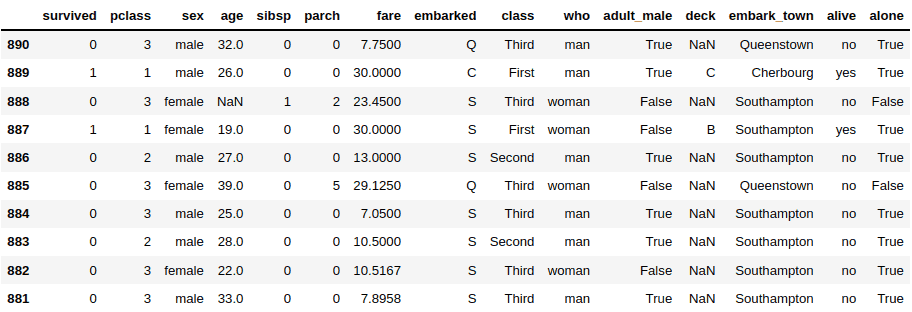I put together a Python Developer Kit with over 100 pre-built Python scripts covering data structures, Pandas, NumPy, Seaborn, machine learning, file processing, web scraping and a whole lot more - and I want you to have it for free. Enter your email address below and I'll send a copy your way.

Yes, I'll take a free Python Developer Kit

## Sorting Dataframes by Column Names

You can also sort a dataframe by the column name so the column names appear in alphabetical order. You need to pass 1 as the value for the “index” parameter of the `sort_index()` function. As a result, a Pandas dataframe is sorted from left to right or right to left in alphabetical order of the column names.

The following script sorts the “titanic” dataset horizontally in ascending order of the column names.

``````temp_df = df.sort_index(axis = 1)

Output: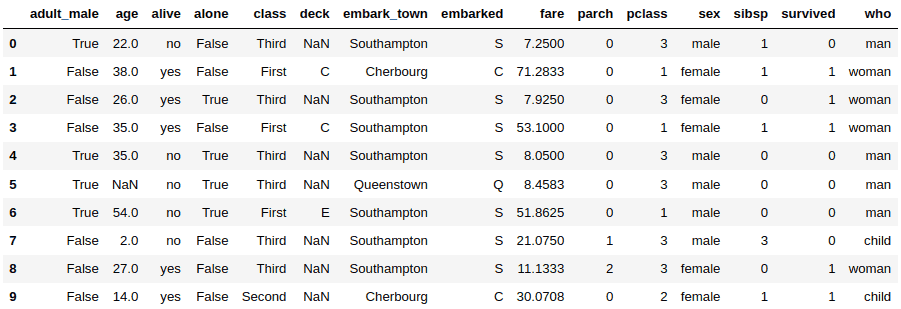Similarly, you can sort a Pandas dataframe horizontally in the reverse order by passing a “False” value to the `ascending` parameter, as shown in the example below.

``````temp_df = df.sort_index(axis = 1, ascending = False)

Output: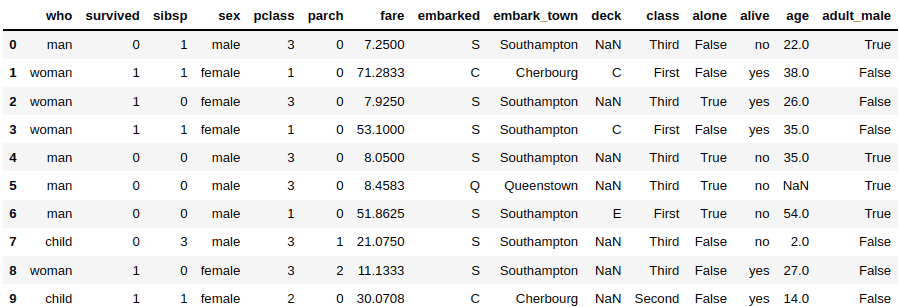## Sorting DataFrame Columns with Missing Values

A Pandas DataFrame may contain missing values. For example, the following script shows that 19.86% of the “age” column contains missing values.

``df.isnull().mean()``

Output: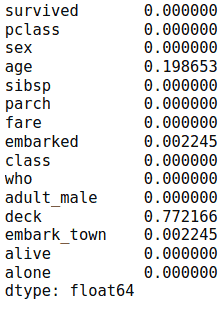By default when you sort a Pandas dataframe, NULL values appear at the end of the sorted dataframe. As an example, the following script displays the last 10 values of the “titanic” dataset sorted by the ascending order of the values in the “age” column. You can see the NaN (null) values at the end.

``````temp_df = df.sort_values('age')
temp_df.tail(10)``````

Output: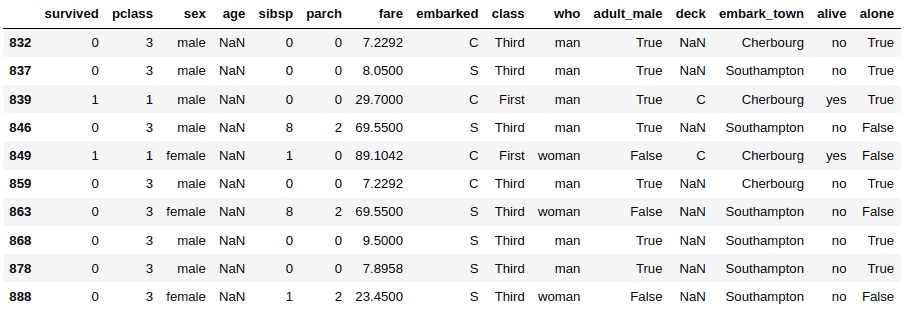If you want null values to appear at the top of a sorted dataframe, you can pass “first” as the value for the `na_position` column.

``````temp_df = df.sort_values('age',
na_position="first")